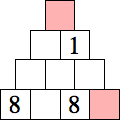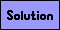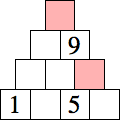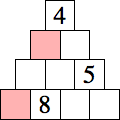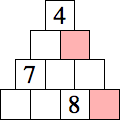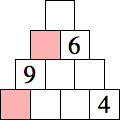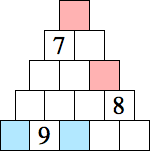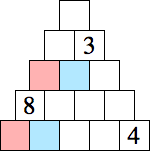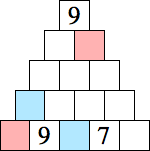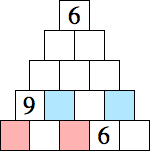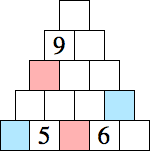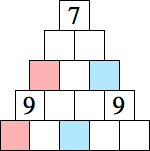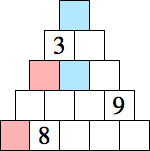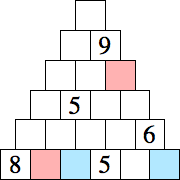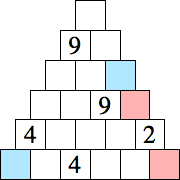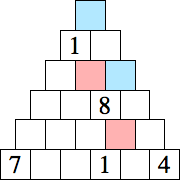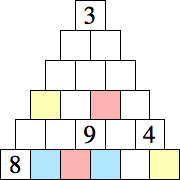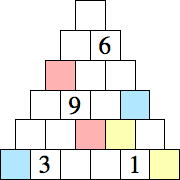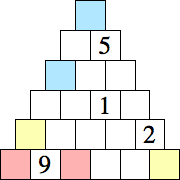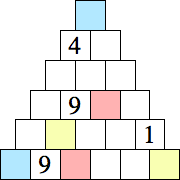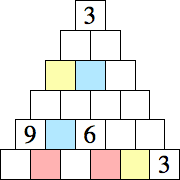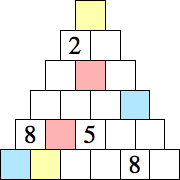Pyramid Puzzles

Insert a number 1-9 in each vacant square so that the number in each square is the sum or difference of the numbers in the two squares immediately below it. Digits in similarly colored squares will be the same. An example is shown below.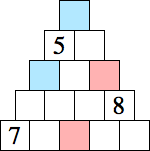→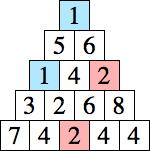Each puzzle below has a unique solution.